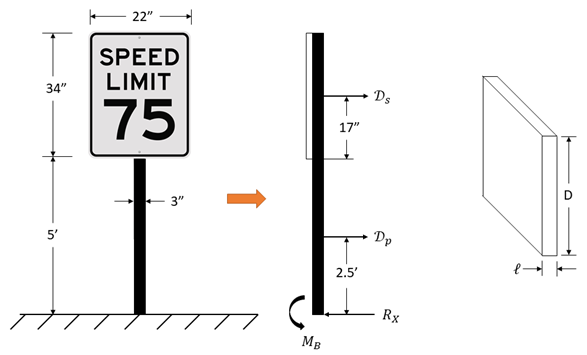## Stop at the Sign

A 22 inch by 34 inch speed limit sign is supported on a 3 inch-wide, 5 ft-long pole. Estimate the bending moment in the pole at ground level when a 30 mph wind blows against the sign. Assume aspect ratio for the sign is less than 0.1. Assume the pole is a square rod with sharp corners. Also assume the density of air is 0.00238 slug/ft^3. Note the drag force coefficient of a squared rod with sharp corners is 2.2.Hint
The drag force on objects immersed in a large body of flowing fluid or objects moving through a stagnant fluid:
$$D=\frac{1}{2}\rho U^{2}C_{D}A$$$where $$C_D$$ is the drag coefficient, $$U$$ is the flowing fluid or moving object’s velocity, $$\rho$$ is the fluid density, and $$A$$ is the projected area of blunt objects with axes perpendicular to the flow. Hint 2 Take the moment about the origin/base of the pole: $$\sum M_{o}=0=Force \times Distance$$$
For equilibrium:
$$\sum M_{origin}=0=M_{B}-M_{P}-M_{s}$$$where $$M_B$$ is the bending moment, $$M_P$$ is the moment on the pole, and $$M_s$$ is the moment on the sign. Remember that $$M=Force \times Distance$$ , so the bending moment can be written as: $$M_{B}=2.5ft\cdot D_{P}+(5+\frac{17}{12})ft\cdot D_{s}$$$
where $$D_P$$ is the drag force on the pole, and $$D_s$$ is the drag force on the sign.

The drag force on objects immersed in a large body of flowing fluid or objects moving through a stagnant fluid:
$$D=\frac{1}{2}\rho U^{2}C_{D}A$$$where $$C_D$$ is the drag coefficient, $$U$$ is the flowing fluid or moving object’s velocity, $$\rho$$ is the fluid density, and $$A$$ is the projected area of blunt objects with axes perpendicular to the flow. For the sign, $$\ell/D< 0.1$$ so, $$C_{D_s}=1.9$$ . The post acts as a squared rod with sharp corners, so $$C_{D_p}=2.2$$ . Thus, with $$U=30mph=44ft/s$$ $$D_s=\frac{1}{2}\rho U^{2}C_{D_s}A_s=\frac{1}{2}(0.00238\frac{slug}{ft^{3}})(44\frac{ft}{s})^2(1.9)(\frac{22\cdot 34}{144}ft^2)=22.7\:lb$$$
$$D_P=\frac{1}{2}\rho U^{2}C_{D_p}A_p=\frac{1}{2}(0.00238\frac{slug}{ft^{3}})(44\frac{ft}{s})^2(2.2)(\frac{3\cdot 5}{12}ft^2)=6.34\:lb$$$Finally, $$M_{B}=2.5ft\cdot (6.37lb)+(5+\frac{17}{12})ft\cdot(22.7lb)=162\:ft\cdot lb$$$
$$162\:ft\cdot lb$$\$